# Electromagnetic Induction Questions and Answers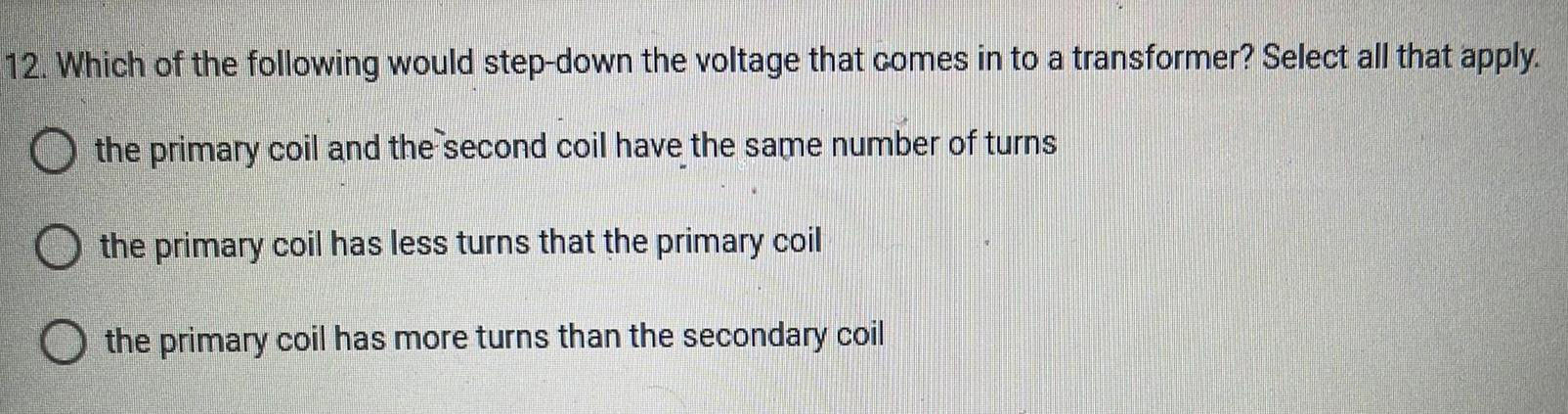Physics
Electromagnetic Induction
12. Which of the following would step-down the voltage that comes in to a transformer? Select all that apply. the primary coil and the second coil have the same number of turns the primary coil has less turns that the primary coil the primary coil has more turns than the secondary coil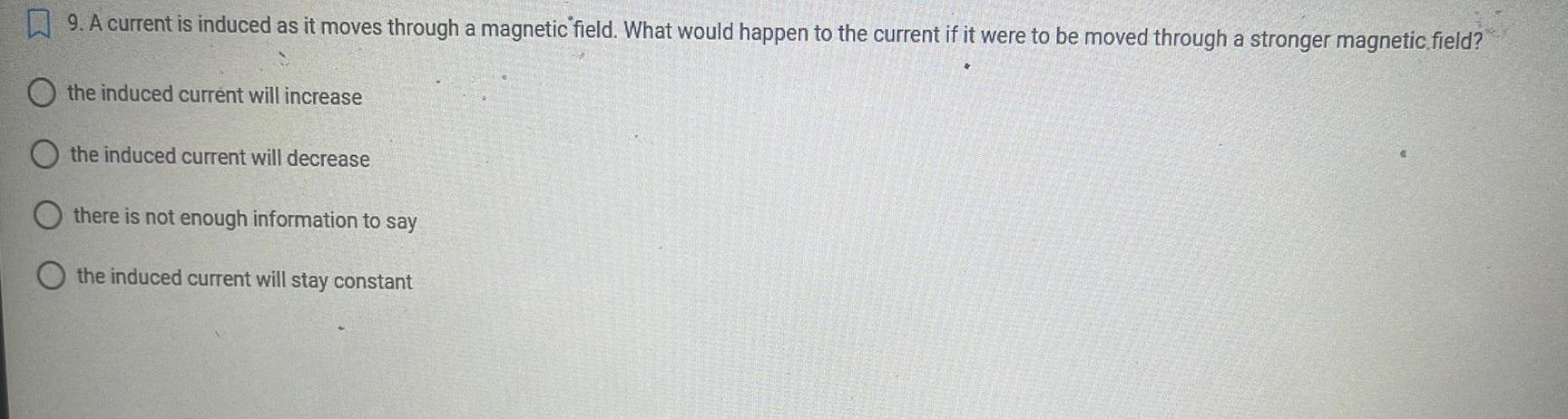Physics
Electromagnetic Induction
9. A current is induced as it moves through a magnetic field. What would happen to the current if it were to be moved through a stronger magnetic field? the induced current will increase the induced current will decrease there is not enough information to say the induced current will stay constant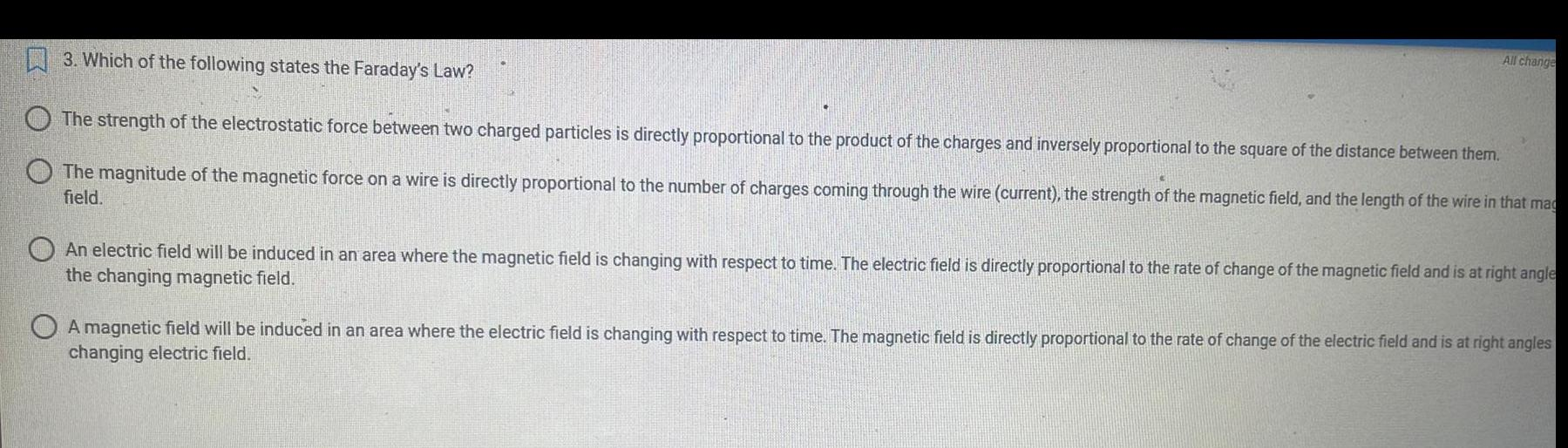Physics
Electromagnetic Induction
3. Which of the following states the Faraday's Law? The strength of the electrostatic force between two charged particles is directly proportional to the product of the charges and inversely proportional to the square of the distance between them. The magnitude of the magnetic force on a wire is directly proportional to the number of charges coming through the wire (current), the strength of the magnetic field, and the length of the wire in that mag field. All change An electric field will be induced in an area where the magnetic field is changing with respect to time. The electric field is directly proportional to the rate of change of the magnetic field and is at right angle the changing magnetic field. A magnetic field will be induced in an area where the electric field is changing with respect to time. The magnetic field is directly proportional to the rate of change of the electric field and is at right angles changing electric field.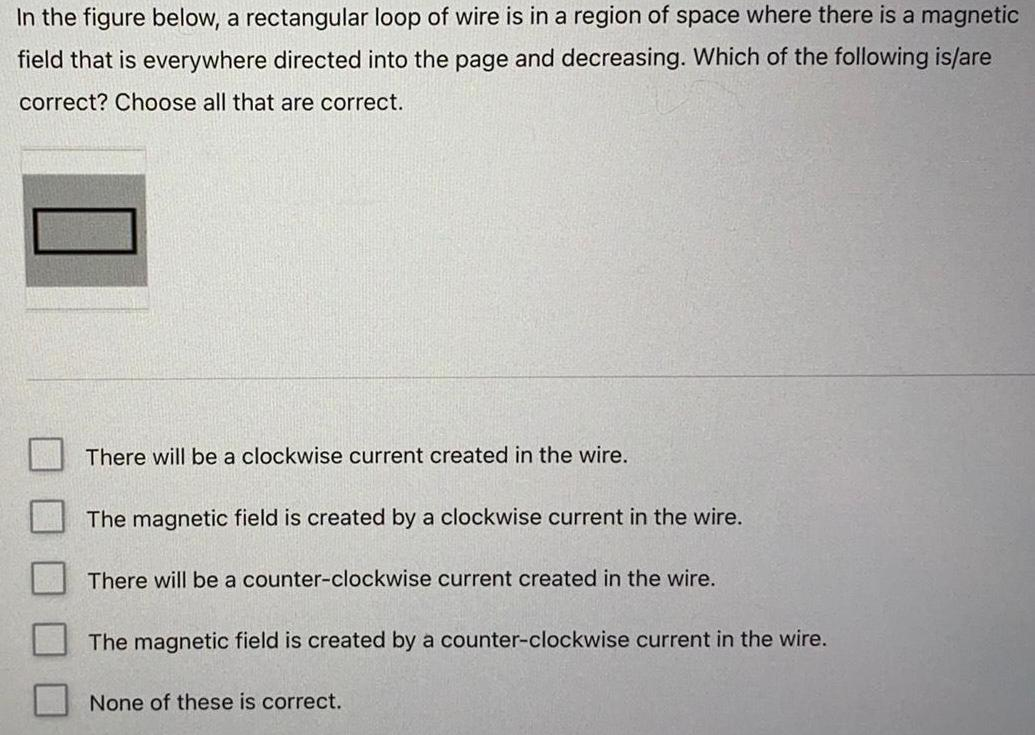Physics
Electromagnetic Induction
In the figure below, a rectangular loop of wire is in a region of space where there is a magnetic field that is everywhere directed into the page and decreasing. Which of the following is/are correct? Choose all that are correct. There will be a clockwise current created in the wire. The magnetic field is created by a clockwise current in the wire. There will be a counter-clockwise current created in the wire. The magnetic field is created by a counter-clockwise current in the wire. None of these is correct.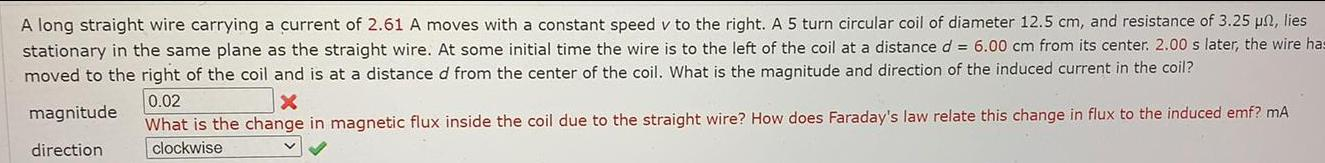Physics
Electromagnetic Induction
A long straight wire carrying a current of 2.61 A moves with a constant speed v to the right. A 5 turn circular coil of diameter 12.5 cm, and resistance of 3.25 un, lies stationary in the same plane as the straight wire. At some initial time the wire is to the left of the coil at a distance d = 6.00 cm from its center. 2.00 s later, the wire has moved to the right of the coil and is at a distance d from the center of the coil. What is the magnitude and direction of the induced current in the coil?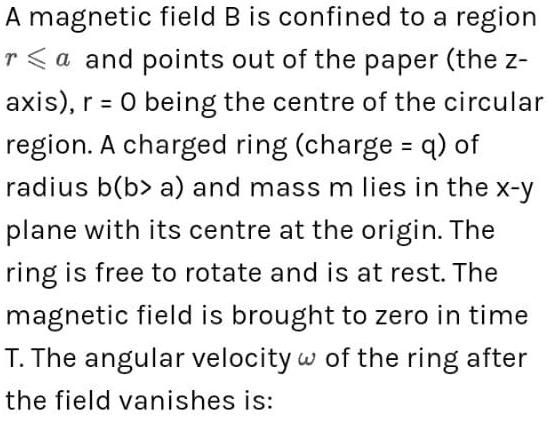Physics
Electromagnetic Induction
A magnetic field B is confined to a region ra and points out of the paper (the z- axis), r = 0 being the centre of the circular region. A charged ring (charge = q) of radius b(b> a) and mass m lies in the x-y plane with its centre at the origin. The ring is free to rotate and is at rest. The magnetic field is brought to zero in time T. The angular velocity w of the ring after the field vanishes is: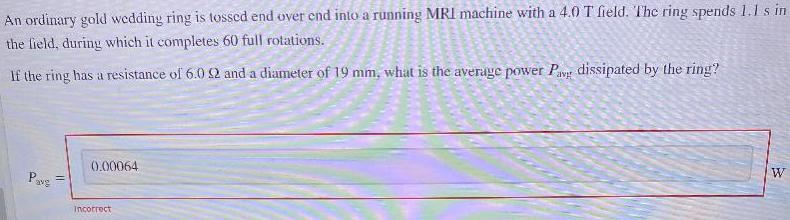Physics
Electromagnetic Induction
An ordinary gold wedding ring is tossed end over end into a running MRI machine with a 4.0 T field. The ring spends 1.1 s in the field, during which it completes 60 full rotations. If the ring has a resistance of 6.0 Ω and a diameter of 19 mm, what is the average power Pav dissipated by the ring?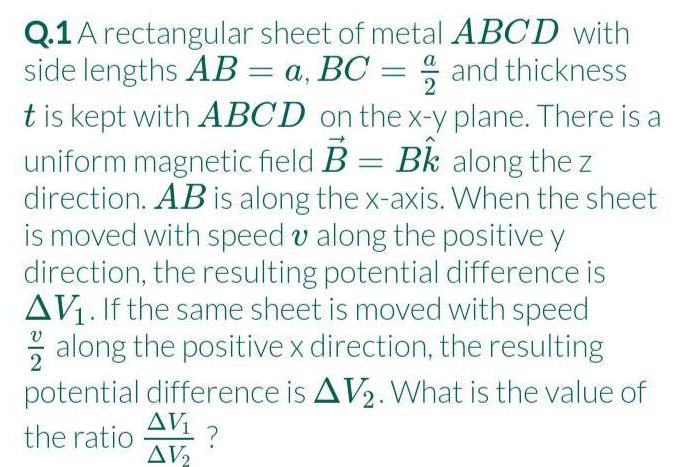Physics
Electromagnetic Induction
A rectangular sheet of metal ABCD with side lengths AB = a, BC = a/2 and thickness t is kept with ABCD on the x-y plane. There is a uniform magnetic field B = Bk along the z direction. AB is along the x-axis. When the sheet is moved with speed v along the positive y direction, the resulting potential difference is ΔV₁. If the same sheet is moved with speed along the positive x direction, the resulting potential difference is ΔV₂. What is the value of the ratioΔV₁/ ΔV₂ ?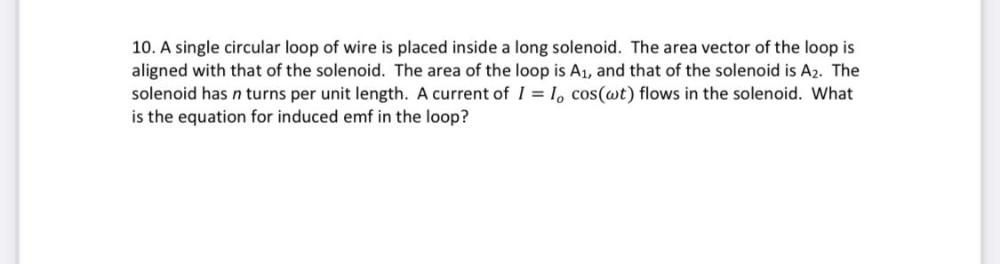Physics
Electromagnetic Induction
A single circular loop of wire is placed inside a long solenoid. The area vector of the loop is aligned with that of the solenoid. The area of the loop is A₁, and that of the solenoid is A₂. The solenoid has n turns per unit length. A current of I = I₀cos(ωt) flows in the solenoid. What is the equation for induced emf in the loop?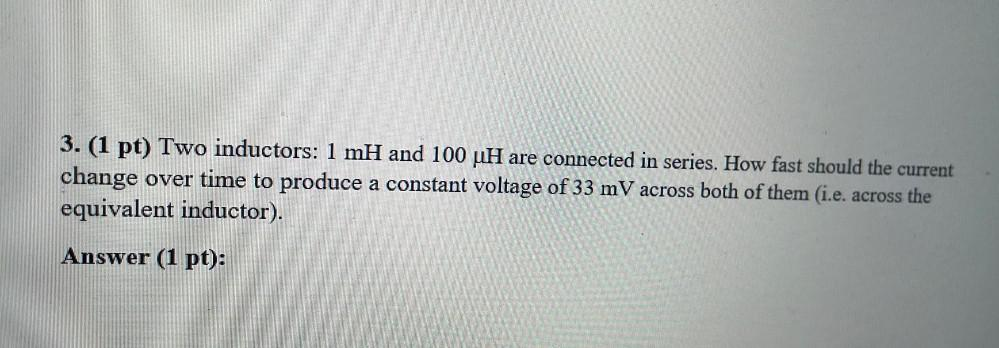Physics
Electromagnetic Induction
Two inductors: 1 mH and 100 μH are connected in series. How fast should the current change over time to produce a constant voltage of 33 mV across both of them (i.e. across the equivalent inductor).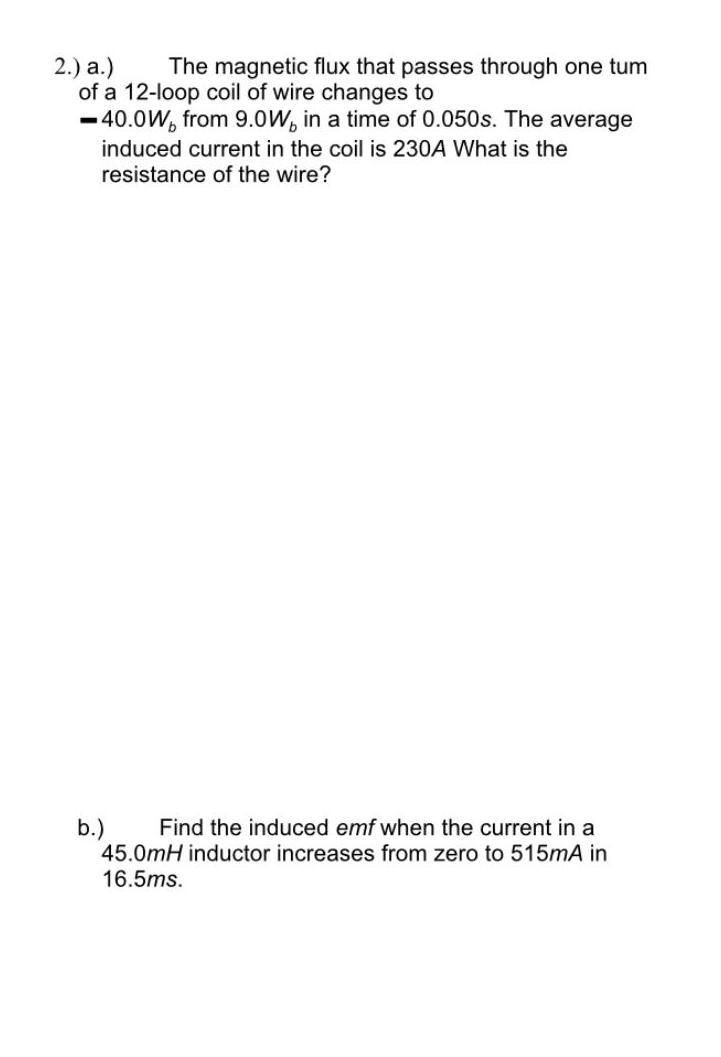Physics
Electromagnetic Induction
The magnetic flux that passes through one tum of a 12-loop coil of wire changes to -40.0W, from 9.0W, in a time of 0.050s. The average induced current in the coil is 230A What is the resistance of the wire? Find the induced emf when the current in a 45.0mH inductor increases from zero to 515mA in 16.5ms.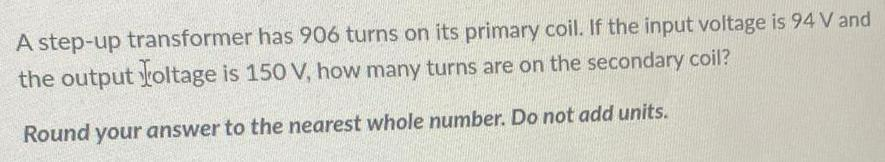Physics
Electromagnetic Induction
A step-up transformer has 906 turns on its primary coil. If the input voltage is 94 V and the output Voltage is 150 V, how many turns are on the secondary coil? Round your answer to the nearest whole number. Do not add units.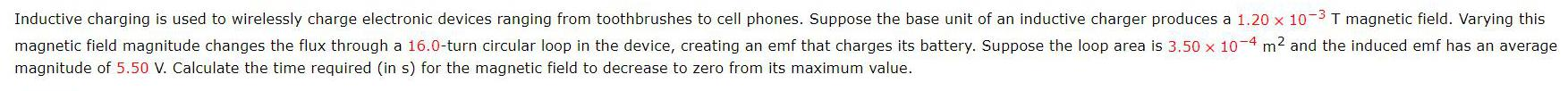Physics
Electromagnetic Induction
Inductive charging is used to wirelessly charge electronic devices ranging from toothbrushes to cell phones. Suppose the base unit of an inductive charger produces a 1.20 x 10^-3 T magnetic field. Varying this magnetic field magnitude changes the flux through a 16.0-turn circular loop in the device, creating an emf that charges its battery. Suppose the loop area is 3.50 x 10^-4 m² and the induced emf has an average magnitude of 5.50 V. Calculate the time required (in s) for the magnetic field to decrease to zero from its maximum value.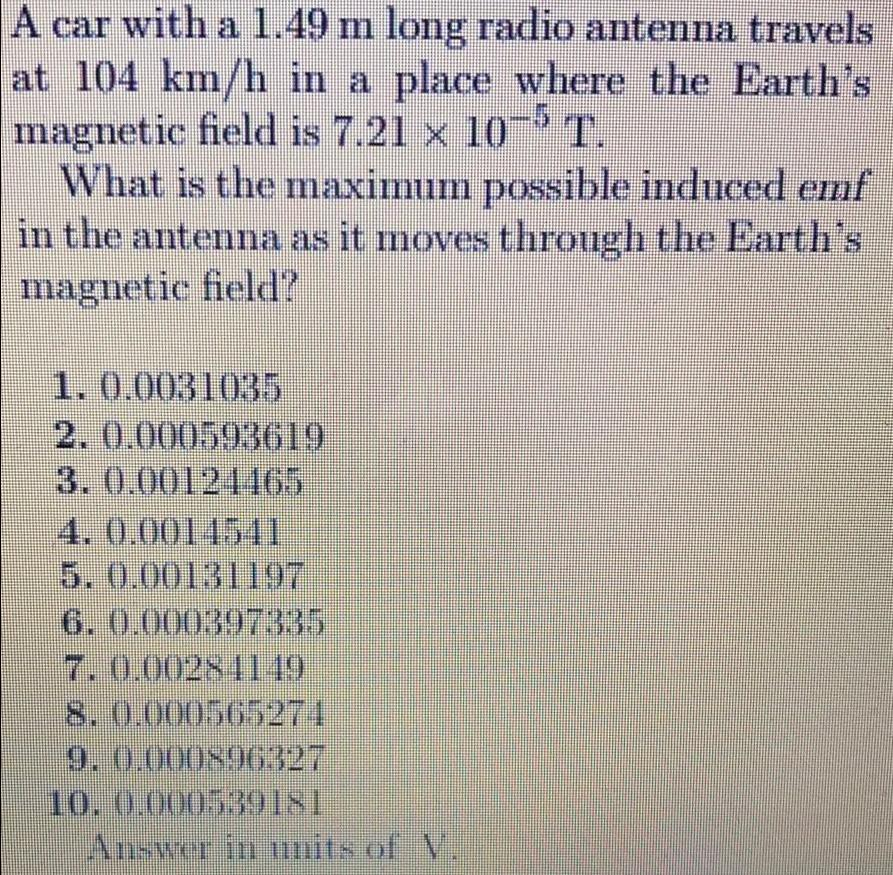Physics
Electromagnetic Induction
A car with a 1.49 m long radio antenna travels at 104 km/h in a place where the Earth's magnetic field is 7.21 × 10−5 T. What is the maximum possible induced emf in the antenna as it moves through the Earth's magnetic field? 1.0.0031035 2. 0.000593619 3. 0.00124465 4. 0.0014541 5. 0.00131197 6. 0.000397335 7.0.00284149 8. 0.000565271 9. 0.000896327 10. 0.000539181 Answer in units of V.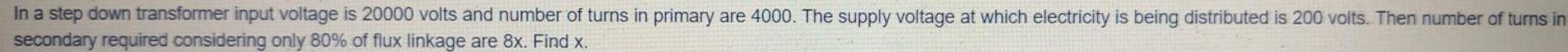Physics
Electromagnetic Induction
In a step down transformer input voltage is 20000 volts and number of turns in primary are 4000. The supply voltage at which electricity is being distributed is 200 volts. Then number of turns in secondary required considering only 80% of flux linkage are 8x. Find x.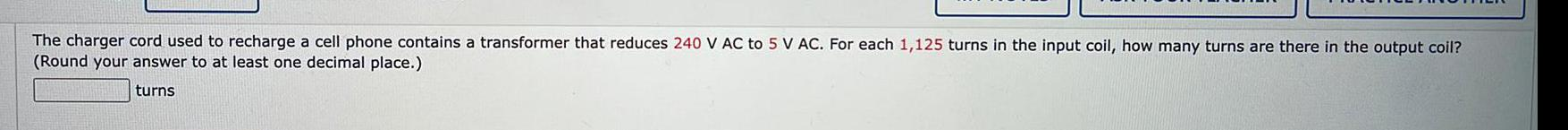Physics
Electromagnetic Induction
The charger cord used to recharge a cell phone contains a transformer that reduces 240 V AC to 5 V AC. For each 1,125 turns in the input coil, how many turns are there in the output coil? (Round your answer to at least one decimal place.)
1